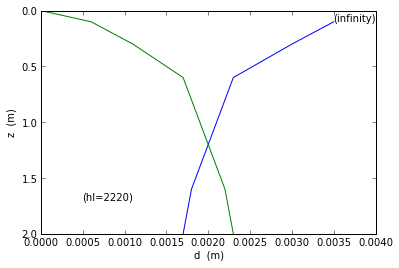# Chapter 10: Condensation and Vaporization Heat Transfer¶

### Example 10.1 Page NO.527¶

In [ ]:
%matplotlib inline

In :
rou_f=0.909*62.4    # density in lbm/ft**3
cp=1.037             # specific heat BTU/(lbm-degree Rankine)
v_f=0.204e-5         # viscosity in ft**2/s
kf=0.393             #  thermal conductivity in BTU/(lbm.ft.degree Rankine)
a=6.70e-3            # diffusivity in ft**2/hr
Pr=1.099             # Prandtl Number
V_v=4.937             # specific volume in ft**3/lbm from superheated steam tables
rou_v=1/V_v           # vapour density
g=32.2
hfg=888.8             # from saturated steam tables
Tg=327.81
Tw=325
L=2.0                 # length in ft
W=3.0                 # width in ft
z=0.204*10**-5         # distance from entry of plate in ft

y=((4*kf*v_f*(Tg-Tw)/3600.0)/(rou_f*g*hfg*(1-(rou_v/rou_f))))**(1/4.0)  #let y=delta/z**(1/4)
hz=1665              #From Table 10.1
hL=(4/3.0)*hz           # at plate end
mf=(hL*L*W*(Tg-Tw))/hfg
q=mf*hfg
Re=(4*mf/3600)/(W*rou_f*v_f)

print"The amount of steam condensed is ",round(mf,1),"lbm/h"
print"The heat transfer rate is ",round(q,0),"BTU/hr"

import matplotlib.pyplot as plt
fig = plt.figure()

x1=[0.0017,0.0018,0.0023,0.0030,0.0035]
z1=[2,1.6,0.6,0.3,0.1]

x2=[0.0023,0.0022,0.0017,0.0011,0.0006,0]
z2=[2,1.6,0.6,0.3,0.1,0]

xlabel("d  (m)")
ylabel("z  (m)")
plt.xlim((0,0.004))
plt.ylim((2,0))

ax.annotate('(infinity)', xy=(0.0035,0.1))
ax.annotate('(hl=2220)', xy=(0.0005,1.7))
a1=plot(x1,z1)
a1=plot(x2,z2)

The amount of steam condensed is  42.1 lbm/h
The heat transfer rate is  37429.0 BTU/hr### Example 10.2 Page NO.532¶

In :
rou_f=974.0   # density in kg/m**3
cp_1=4196.0   # specific heat in J/(kg*K)
v_1=0.364e-6  # viscosity in m**2/s
Pr_1=2.22     # Prandtl Number
kf=0.668     # thermal conductivity in W/(m.K)
a_1=1.636e-7 # diffusivity in m**2/s
Vv=1.9364     # specific volume in m**3/kg
rou_v=1/Vv    # vapor density
g=9.81
hfg=2257.06*1000
Tg=100
Tw=60
L=1

OD=0.03340
hD=0.782*((g*rou_f*(1-(rou_v/rou_f))*(kf**3)*hfg)/(v_1*OD*(Tg-Tw)))**(1/4.0)
hD=10720       #According to the book
import math
q=hD*math.pi*OD*L*(Tg-Tw)
mf=q/hfg

print"The heat flow rate is ",round(q,0),"W"
print"The rate at which steam condenses is ",round(mf*3600,0),"kg/hr"

The heat flow rate is  44994.0 W
The rate at which steam condenses is  72.0 kg/hr


### Example 10.3 Page NO. 538¶

In :
rou_f=958            # density in kg/m**3
cp_f= 4217           # specific heat in J/(kg*K)
v_f= 2.91e-7         # viscosity in m**2/s
Pr_f =1.76           # Prandtl Number
rou_g=0.596
sigma=0.0589         # surface tension in N/m
hfg=2257000
Tw=120.0
Tg=100.0
D=.141               # diameter of pan in m
g=9.81
gc=1

Cw=0.0132           # formechanically polished stainless steel from table 10.2
q_A=(rou_f*v_f*hfg)*((g*rou_f*(1-(rou_g/rou_f)))/(sigma*gc))**(0.5)*((cp_f*(Tw-Tg))/(Cw*hfg*Pr_f**1.7))**3
A=math.pi*D**2/4.0
p=q_A*A               # power delivered to the water in W
mf=q/hfg              # water evaporation rate
q_cr=0.18*hfg*(sigma*g*gc*rou_f*rou_g**2)**(0.25)

print"(a)The power delivered to the water is  kW",round(q/1000,2),"KW"
print"(b)The water evaporation rate is ",round(mf*3600,2),"kg/h"
print"(c)The critical heat flux is ",round(q_cr,0),"W/sq.m"

(a)The power delivered to the water is  kW 4.98 KW
(b)The water evaporation rate is  7.94 kg/h
(c)The critical heat flux is  1521299.0 W/sq.m

In [ ]: